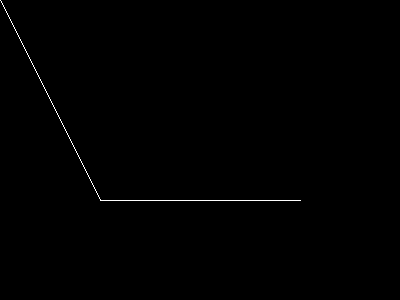PHP 8.1.0 Released!

# imageopenpolygon

(PHP 7 >= 7.2.0, PHP 8)

imageopenpolygonDraws an open polygon

### Descripción

Signature as of PHP 8.0.0 (not supported with named arguments)

imageopenpolygon(GdImage `\$image`, array `\$points`, int `\$color`): bool

Alternative signature (deprecated as of PHP 8.1.0)

imageopenpolygon(
GdImage `\$image`,
array `\$points`,
int `\$num_points`,
int `\$color`
): bool

imageopenpolygon() draws an open polygon on the given `image`. Contrary to imagepolygon(), no line is drawn between the last and the first point.

### Parámetros

``` image```

Un recurso image, es devuelto por una de las funciones de creación de imágenes, como imagecreatetruecolor().

`points`

An array containing the polygon's vertices, e.g.:

 points = x0 points = y0 points = x1 points = y1

`num_points`

Total number of points (vertices), which must be at least 3.

If this parameter is omitted as per the second signature, `points` must have an even number of elements, and `num_points` is assumed to be `count(\$points)/2`.
`color`

Un identificador de color creato con imagecolorallocate().

### Valores devueltos

Devuelve `true` en caso de éxito o `false` en caso de error.

### Historial de cambios

Versión Descripción
8.1.0 The parameter `num_points` has been deprecated.
8.0.0 `image` expects a GdImage instance now; previously, a resource was expected.

### Ejemplos

Ejemplo #1 imageopenpolygon() example

``` <?php// Create a blank image\$image = imagecreatetruecolor(400, 300);// Allocate a color for the polygon\$col_poly = imagecolorallocate(\$image, 255, 255, 255);// Draw the polygonimageopenpolygon(\$image, array(        0,   0,        100, 200,        300, 200    ),    3,    \$col_poly);// Output the picture to the browserheader('Content-type: image/png');imagepng(\$image);imagedestroy(\$image);?> ```

El resultado del ejemplo sería algo similar a:### Ver tambiénadd a note

### User Contributed Notes 1 note

-1
marco at oostende dot nl
2 years ago
``` In case you want to use an open polygon but are stuck with a PHP version prior to 7.2, a solution may be to 'backplot' your array to its original start. Say you have an array of pixels (below seperated by commas)<?php\$arr = array();for (\$i = 0; \$i < count(\$pixels); \$i++) {    \$pixel = explode(',', \$pixels[\$i]);    if ((\$pixel > 0) && (\$pixel > 0)) {        \$arr[] = \$pixel;        \$arr[] = \$pixel;    }}imagepolygon(\$im, \$arr, (count(\$arr) / 2), \$otcolor);?>you can replace this by something like<?php\$arr = array();for (\$i = 0; \$i < count(\$pixels); \$i++) {    \$pixel = explode(',', \$pixels[\$i]);    \$arr[] = \$pixel;    \$arr[] = \$pixel;}// imageopenpolygon(\$im, \$arr, (count(\$arr) / 2), \$otcolor) is not possible, so...for (\$i = (count(\$pixels)-1); \$i >= 0; \$i--) {    \$pixel = explode(',', \$pixels[\$i]);    \$arr[] = \$pixel;    \$arr[] = \$pixel;}imagepolygon(\$im, \$arr, (count(\$arr) / 2), \$otcolor);?> ```